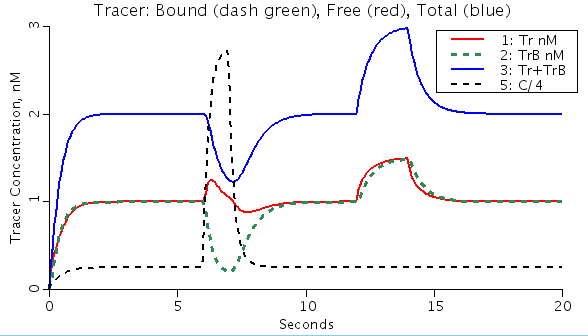# Comp1Flow.TracerBind

Model number
0110

Tracer and Mother solute flow past a binding site. Equilibrium upset by an added pulse of mother solute, dislodging tracer.

## Description

```One compartment model of mother solute C and tracer labeled C, called Tr,
with slow binding to a binding ligand B os constant total, Btotal, and
which does not leave the flowing region..Volume V is constant. Total
concentration of binding ligand, Btotal = unbound B and the complex CB.
Tracer and mother substance flow in and out. Concn of unbound ligand is
determined by the concns of mother and tracer analytes relative to the
Kd mM, which is the dissociation constant for ligand-solute binding.
The purpose of this model is to demonstrate that when the concn of
mother substance is raised, tracer that has been attached to a binding
ligand is driven off and washed out. The function generator fgen_1 is
set up to provide a constant infusion of mother substance at 1 mM to
which an additional 1-second pulse is added at t = 6 seconds. The tracer
infusion is constant at 1 nM through to 12 seconds. The concentrations and
the Kd are chosen ao that the pulse of mother solute suddenly reduces the
amount of free ligand, and competes with the tracer that is already
attached, causing a transient release of tracer and a sudden diminution
in the total amount of tracer present in the mixing chamber. An external
detector would give a signal proportional to Tr + TrB, the total tracer
present.
At 12 seconds a 2 second pulse of additional tracer is added, but if
at a tracer level it has no effect on the mother substance binding.
Explore the influence of making this pulse large by changing "p2amplitude"
for Pulse 2 in fgen_2.

Constants:
Btotal = total concentration of binding ligand, mmol/ml
V = Volume of compartment, ml
kon = binding rate, 1/mmol*sec
koff = off rate, 1/sec

Variables:
C(t) = Concentration of mother analyte, mmol/ml
Tr(t) = Concentration of tracer analyte, nmol/ml
CB(t) = Concentration of bound mother analyte with ligand B, mmol/ml
TrB(t) = Concentration of bound tracer with ligand B, nmol/ml
free Ligand [B] = [Btotal]- [CB]-[TrB]```Figure: Effect of large increase of mother solute (Dashed black, C/4) on bound tracer (Dashed green, TrB) concentration. Tracer is driven off binding sites. Assumes binding ligand conc is fixed.

## Equations

The equations for this model may be viewed by running the JSim model applet and clicking on the Source tab at the bottom left of JSim's Run Time graphical user interface. The equations are written in JSim's Mathematical Modeling Language (MML). See the Introduction to MML and the MML Reference Manual. Additional documentation for MML can be found by using the search option at the Physiome home page.

References
```Bassingthwaighte JB and Goresky CA. Modeling in the analysis of solute and
water exchange in the  microvasculature. In: Handbook of Physiology.
Sect. 2, The Cardiovascular System. Vol IV, The Microcirculation, edited by
Renkin EM and Michel CC. Bethesda, MD: Am. Physiol. Soc., 1984, pp 549-626.
( See Permeation p.560.)```
Key terms
Tracer
mother
analyte binding to fixed ligand
dissociation curves
Tutorial
competitive binding
dissociation and association rates
equilibrium